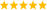# LabVIEW

cancel
Showing results for
Did you mean:

Highlighted

## How to calculate the angle of the next turn

Hi,

I am currently working on a project which involves using a set of coordinates to control a car. At the moment, these waypoints are input into an array of x and y  and the car is commanded to traverse the line between them.

The problem i have is I cannot work out how, given 3 points, A (being the previous waypoint), B (being the waypoint the car is approaching) and C (being the next waypoint the car will be travelling towards after it has turned) I can calculate whether the car is turning right or left at B.

Thanks, Luc

Message 1 of 8
(1,268 Views)

## Re: How to calculate the angle of the next turn

You could calculate the slope between A and B and the slope between B and C.  If the slope between B and C is greater than the slope between A and B it is a left turn and vice-versa.
Message 2 of 8
(1,264 Views)

## Re: How to calculate the angle of the next turn

It might be easier to do all that using complex numbers (RE,IM) instead of number pairs (X,Y). You can get the difference in vector angle, which you then need to fold into a unique range (e.g. 0..360 or -180..180). Half of the range is "right", and the other half is "left".

See how far you get. 😉LabVIEW Champion. It all comes together inGCentralMessage 3 of 8
(1,258 Views)

## Re: How to calculate the angle of the next turn

I realized after I posted that slope alone will not be good enough because you also need a positive/negative direction.  You will need to create a heading (0 - 360 degrees) from your x-y coordinates for the A-B path and then for the B-C path.  It takes a fair amount of math, but if the second heading is  anywhere from 0 to -180 from the first heading it is a left turn. If the difference in heading is between 0 and 180 from the first heading to the second heading, it will be a right turn.
Message 4 of 8
(1,257 Views)

## Re: How to calculate the angle of the next turn

That is what i thought, but the problem arises in the fact that it could be travelling either way down a line of the same gradient and therefore this does not give a reliable prediction.
Message 5 of 8
(1,256 Views)

## Re: How to calculate the angle of the next turn

I have now connected it up so that the differences for A->B (x and y) go into the Re and Im of one complex number, and the same for B->C. Can i then just convert these into polar and compare theta?
Message 6 of 8
(1,239 Views)

## Re: How to calculate the angle of the next turn

Hi Luc,

yesOr you calc the difference of theta...

Best regards,
GerdW
CLAD expired, using LV2011SP1 + LV2017 (+LV2019 sometimes) on Win7+cRIO
Kudos are welcomeMessage 7 of 8
(1,214 Views)

## Re: How to calculate the angle of the next turn

You can simply divide the two complex numbers and the theta of the result will be the angle you want. 😉LabVIEW Champion. It all comes together inGCentralMessage 8 of 8
(1,205 Views)